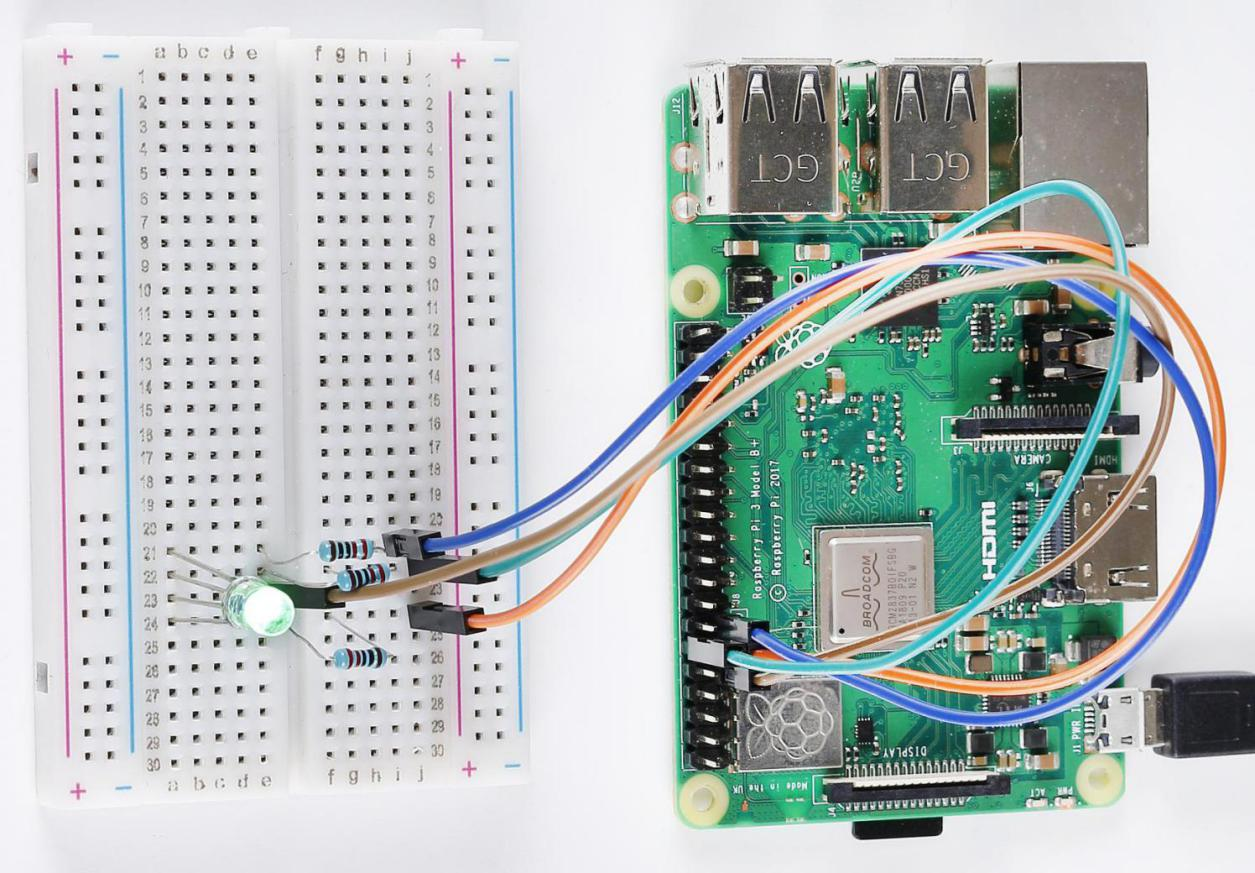# Lesson 4 RGB LED¶

## Introduction¶

Previously we’ve used the PWM technology to control an LED’s brightness. In this lesson, we will use it to control an RGB LED to flash various kinds of colors.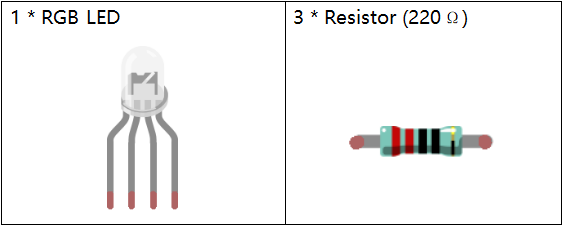## Principle¶

RGB LED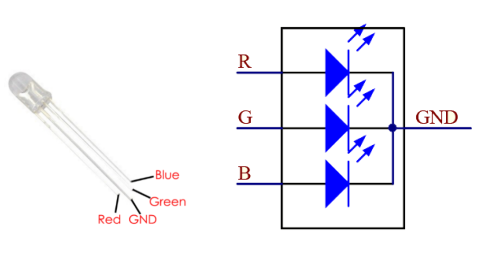The three primary colors of the RGB LED can be mixed into various colors by brightness. The brightness of LED can be adjusted with PWM. Raspberry Pi has only one channel for hardware PWM output, but it needs three channels to control the RGB LED, which means it is difficult to control the RGB LED with the hardware PWM of Raspberry Pi. Fortunately, the softPwm library simulates PWM (softPwm) by programming. You only need to include the header file softPwm.h (for C language users), and then call the API it provides to easily control the RGB LED by multi-channel PWM output, so as to display all kinds of color.

## Schematic Diagram¶

After connecting the pins of R, G, and B to a current limiting resistor, connect them to the pin 11, pin 12, and pin 13 respectively. The longest pin (GND) of the LED connects to the GND of the Raspberry Pi. When the three pins are given different PWM values, the RGB LED will display different colors.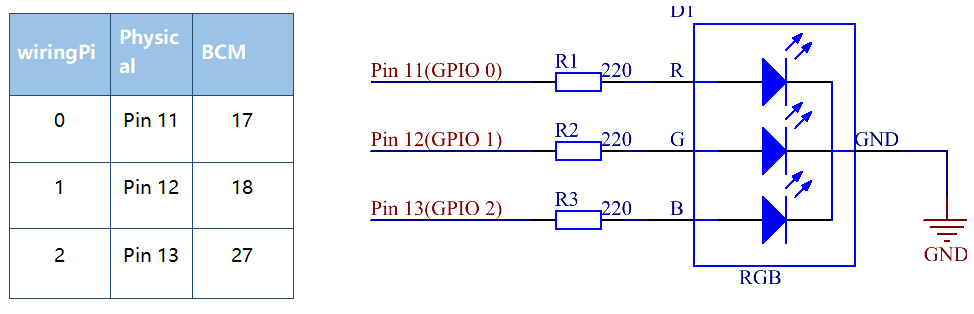## Build the Circuit¶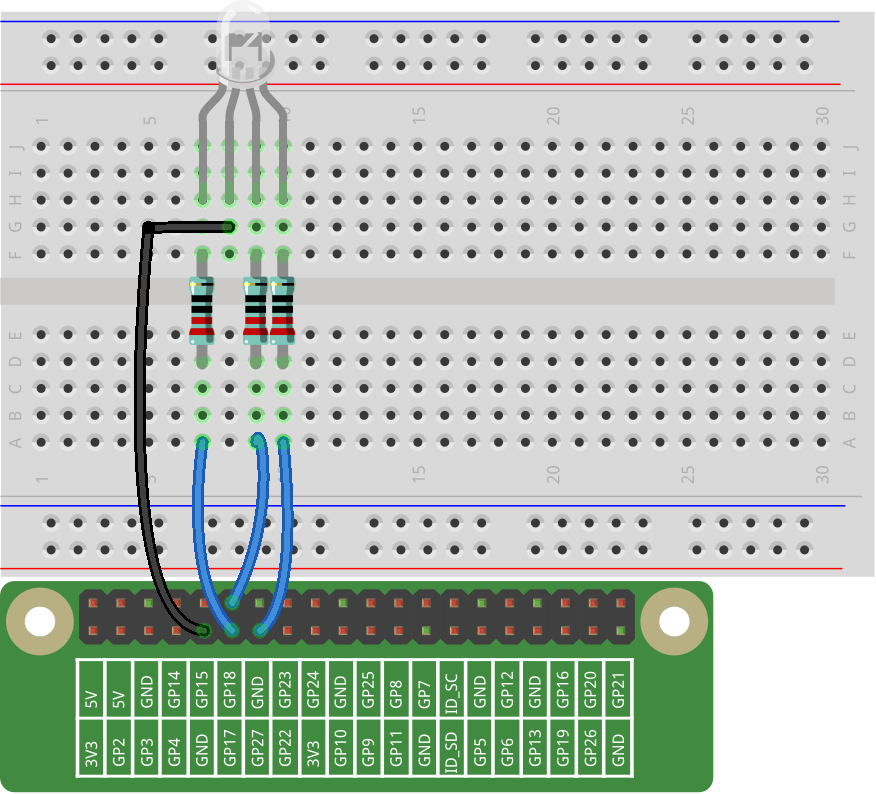## For C Language Users¶

### Command¶

1. Go to the folder of the code.

```cd /home/pi/electronic-kit/for-raspberry-pi/c/Lesson_4_RGBLed
```

2. Compile the code.

```gcc 4_rgbLed.c -lwiringPi
```

3. Run the executable file.

```sudo ./a.out
```

After the code runs, you will see that RGB displays red, green, blue, yellow, pink, and cyan.

Note

If it does not work after running, or there is an error prompt: “wiringPi.h: No such file or directory”, please refer to C code is not working?.

### Code¶

```#include <wiringPi.h>
#include <softPwm.h>
#include <stdio.h>

#define uchar unsigned char

#define LedPinRed    0
#define LedPinGreen  1
#define LedPinBlue   2

// define function used for initializing I/O port to output for pwm.
void ledInit(void){
softPwmCreate(LedPinRed,  0, 100);
softPwmCreate(LedPinGreen,0, 100);
softPwmCreate(LedPinBlue, 0, 100);
}

void ledColorSet(uchar r_val, uchar g_val, uchar b_val){
softPwmWrite(LedPinRed,   r_val);
softPwmWrite(LedPinGreen, g_val);
softPwmWrite(LedPinBlue,  b_val);
}

int main(void){
if(wiringPiSetup() == -1){ //when initialize wiring failed, printf message to screen
printf("setup wiringPi failed !");
return 1;
}

ledInit();

while(1){
printf("Red\n");
ledColorSet(0xff,0x00,0x00);   //red
delay(500);
printf("Green\n");
ledColorSet(0x00,0xff,0x00);   //green
delay(500);
printf("Blue\n");
ledColorSet(0x00,0x00,0xff);   //blue
delay(500);
printf("Yellow\n");
ledColorSet(0xff,0xff,0x00);   //yellow
delay(500);
printf("Purple\n");
ledColorSet(0xff,0x00,0xff);   //purple
delay(500);
printf("Cyan\n");
ledColorSet(0xc0,0xff,0x3e);   //cyan
delay(500);
}

return 0;
}
```

### Code Explanation¶

```12.void ledInit(void){
13.    softPwmCreate(LedPinRed,  0, 100);
14.    softPwmCreate(LedPinGreen,0, 100);
15.    softPwmCreate(LedPinBlue, 0, 100);
16.}
```

Create a function to set the LedPinRedLedPinGreen and LedPinBlue as PWM pins, then set their period between 0x100us-100x100us.

The prototype of the function softPwmCreate(LedPinRed, 0, 100) is as follows：

```int softPwmCreate(int pin,int initialValue,int pwmRange);
```

pin: Any GPIO pin of Raspberry Pi can be set as a PWM pin.

initialValue: The initial pulse width is that initialValue times100us.

pwmRange: the period of PWM is that pwmRange times100us.

```18.void ledColorSet(uchar r_val, uchar g_val, uchar b_val){
19.    softPwmWrite(LedPinRed,   r_val);
20.    softPwmWrite(LedPinGreen, g_val);
21.    softPwmWrite(LedPinBlue,  b_val);
22.}
```

This function is to set the colors of the LED. Using RGB, the formal parameter r_val represents the luminance of the red one, g_val of the green one, b_val of the blue one.

The prototype of the function softPwmWrite(LedPinBlue, b_val) is as follows：

```void softPwmWrite (int pin, int value) ;
```

pin: Any GPIO pin of Raspberry Pi can be set as a PWM pin.

Value: The pulse width of PWM is value times 100us. Note that value can only be less than pwmRange defined previously, if it is larger than pwmRange, the value will be given a fixed value, pwmRange.

```30.    ledInit();
```

Call the ledInit() function in the main function to initialize the LED.

```34.    ledColorSet(0xff,0x00,0x00);   //red
```

Call the function defined before. Write 0xff into LedPinRed and 0x00 into LedPinGreen and LedPinBlue.

Only the Red LED lights up after running this code. If you want to light up LEDs in other colors, just modify the parameters.

## For Python Language Users¶

### Command¶

1. Go to the folder of the code.

```cd /home/pi/electronic-kit/for-raspberry-pi/python
```

2. Run the code.

```sudo python3 4_rgbLed.py
```

After the code runs, you will see that RGB displays red, green, blue, yellow, pink, and cyan.

### Code¶

Note

You can Modify/Reset/Copy/Run/Stop the code below. But before that, you need to go to source code path like `electronic-kit/for-raspberry-pi/python`. After modifying the code, you can run it directly to see the effect.

```import RPi.GPIO as GPIO
import time

COLOR = [0xFF0000, 0x00FF00, 0x0000FF, 0xFFFF00, 0xFF00FF, 0x00FFFF]
pins = {'Red':17, 'Green':18, 'Blue':27}

def setup():
global p_R, p_G, p_B
GPIO.setmode(GPIO.BCM)
for i in pins:
GPIO.setup(pins[i], GPIO.OUT, initial=GPIO.LOW)

# Set all led as pwm channel and frequece to 2KHz
p_R = GPIO.PWM(pins['Red'], 2000)
p_G = GPIO.PWM(pins['Green'], 2000)
p_B = GPIO.PWM(pins['Blue'], 2000)

# Set all begin with value 0
p_R.start(0)
p_G.start(0)
p_B.start(0)

def MAP(x, in_min, in_max, out_min, out_max):
return (x - in_min) * (out_max - out_min) / (in_max - in_min) + out_min

def setColor(color):
# Devide colors from 'color' veriable
R_val = (color & 0xFF0000) >> 16
G_val = (color & 0x00FF00) >> 8
B_val = (color & 0x0000FF) >> 0

# Map color value from 0~255 to 0~100
R_val = MAP(R_val, 0, 255, 0, 100)
G_val = MAP(G_val, 0, 255, 0, 100)
B_val = MAP(B_val, 0, 255, 0, 100)

# Change the colors
p_R.ChangeDutyCycle(R_val)
p_G.ChangeDutyCycle(G_val)
p_B.ChangeDutyCycle(B_val)

print ("color_msg: R_val = %s,  G_val = %s, B_val = %s"%(R_val, G_val, B_val))

def main():
while True:
for color in COLOR:
setColor(color)
time.sleep(0.5)

def destroy():
# Stop all pwm channel
p_R.stop()
p_G.stop()
p_B.stop()
# Turn off all LEDs
GPIO.output(pins, GPIO.LOW)
# Release resource
GPIO.cleanup()

# If run this script directly, do:
if __name__ == '__main__':
setup()
try:
main()
# When 'Ctrl+C' is pressed, the child program
# destroy() will be  executed.
except KeyboardInterrupt:
destroy()
```

### Code Explanation¶

```14.    p_R = GPIO.PWM(pins['Red'], 2000)
```

This statement is used to set the pin to a specific PWM frequency, in this case 2000 Hz.

```23.def MAP(x, in_min, in_max, out_min, out_max):
24.    return (x - in_min) * (out_max - out_min) / (in_max - in_min) + out_min
```

Define a map function for mapping values. For instance, x=50, in_min=0, in_max=255, out_min=0, out_max=100. After the map function mapping, it returns (50-0) * (100-0)/(255-0) +0=19.6, meaning that 50 in 0-255 equals 19.6 in 0-100.

```26.def setColor(color):
27.    R_val = (color & 0xFF0000) >> 16
28.    G_val = (color & 0x00FF00) >> 8
29.    B_val = (color & 0x0000FF) >> 0
```

Create a setColor() function to assign different value to the three variables: R_val, G_val, B_val. Input color should be hexadecimal with red value, blue value, green value. Assign the first two values of the hexadecimal to R_val, the middle two to G_val, assign the last two values to B_val, please refer to the shift operation of the hexadecimal for details. For example, color=0xFF00FF, then R_val=(0xFF00FF & 0xFF0000)>>16 = 0xFF, G_val = 0x00, B_val=0xFF.

```32.# Map color value from 0~255 to 0~100
33.    R_val = MAP(R_val, 0, 255, 0, 100)
34.    G_val = MAP(G_val, 0, 255, 0, 100)
35.    B_val = MAP(B_val, 0, 255, 0, 100)
36.
37.    # Change the colors
38.    p_R.ChangeDutyCycle(R_val)
39.    p_G.ChangeDutyCycle(G_val)
40.    p_B.ChangeDutyCycle(B_val)
```

Map the RGB value from 0 - 255 to 0 - 100. After that, get a value. Then set it to be the duty cycle of R_val, G_val and B_val, and the RGB LED displays corresponding colors.

```46.            for color in COLOR:
47.            setColor(color)
48.            time.sleep(0.5)
```

Assign every item in the COLOR list to the color respectively and change the color of the RGB LED via the setColor() function.

## Phenomenon Picture¶